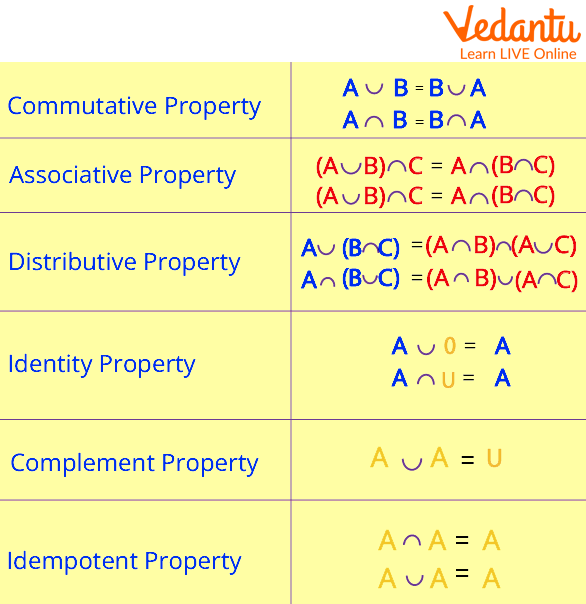Courses
Courses for Kids
Free study material
Free LIVE classes
More

# Properties of SetsLIVE
Join Vedantu’s FREE Mastercalss

## Introduction to Set Properties

A set contains elements or members that can be mathematical objects of any kind, including numbers, symbols, points in space, lines, other geometric shapes, variables, or even other sets. Set properties make it simple to execute many operations on sets. A set is a mathematical model for a collection of various things. Numerous properties, including commutative and associative qualities, are comparable to those of real numbers.

With the help of examples and frequently asked questions, let's learn more about the properties of the union of sets, the intersection of sets, and the complement of sets.

## Properties of Sets

The traits of real numbers apply to sets as well. The three most important properties of sets are the associative property, the commutative property, and the other important property. The following are the formulas for the three sets of qualities, A, B, and C.Properties of Sets

Using the above-mentioned set attributes, it is simple to conduct the various operations of the union of sets, the intersection of sets, and the complement of sets for the supplied sets.

## Properties of Set Operations

A collection of well-defined items is referred to as a set in mathematics. A set's elements remain unchanged if the order in which they are written is changed. A set remains the same if one or more of its components are repeated. We shall discover the crucial characteristics of set operations in this essay.

1. Closure Property

2. Associative Property

3. Commutative Property

5. Identity Property

## Conclusion

Sets have the same qualities as real numbers. Sets have the same associative property, commutative property, and other properties as numbers. The six essential properties of sets are commutative property, associative property, distributive property, identity property, complement property, and idempotent property.

## Properties of Sets Problems

Example 1: Discover the complement of a set A and demonstrate that it complies with the double complement characteristic of sets. Given $A = 1,2,4,5$ and $\mu = 1,2,3,4,5,6,7,8,9,10$.

Solution: $A = 1,2,4,5$and $\mu = 1,2,3,4,5,6,7,8,9,10$ are the given sets.

The objective is to demonstrate the double complement of sets $\left( {A'} \right]' = A$ attribute.

$\begin{array}{l}A = \left\{ {1,2,4,5,7} \right\}\\A' = \mu - A = \left\{ {3,6,8,9,10} \right\}\\\left( {A'} \right)' = \mu - A' = \left\{ {1,2,4,5,7} \right\}\end{array}$

Since the set $\left( {A'} \right)'$ above is identical to the supplied set A, we can conclude that$\left( {A'} \right)' = A$.

As a result, the set in question adheres to the double complement feature of sets.

Example 2: Find the union of sets A and B, then demonstrate that it complies with the union of sets' commutative property. $A = 1,2,3,4,5,6{\rm{ and }}B = 2,3,5,7,8,9$ are given.

Solution: The given sets are$A = 1,2,3,4,5,6{\rm{ and }}B = 2,3,5,7,8,9$.

$A \cup B = B \cup A$ is the commutative property of a union of sets.

$A \cup B = \left\{ {1,2,3,4,5,6,7,8,9} \right\}$

$B \cup A = \left\{ {1,2,3,4,5,6,7,8,9} \right\}$ . We can see from the two sets above that$A \cup B = B \cup A$.

As a result, the commutative property of the union of sets is observed in the two specified sets.

Last updated date: 30th Sep 2023
Total views: 78k
Views today: 0.78k

## FAQs on Properties of Sets

1. What 5 categories of sets are there?

Some different kinds of sets include the infinite set, the finite set, the equivalent set, the subset, the universal set, and the superset.

2. What Purpose Do Set Properties Serve?

Numerous operations can be carried out across sets using the features of sets. The qualities of sets can be used to conveniently carry out the operations of union, intersection, and complement.

3. What Qualifies Sets Of Equal Sets As Sets?

The elements in both sets are identical, and both equal sets include the same number of elements. Equal sets have equal cardinality.